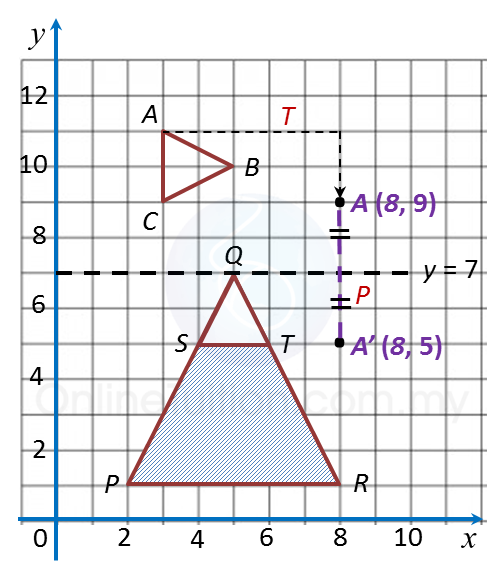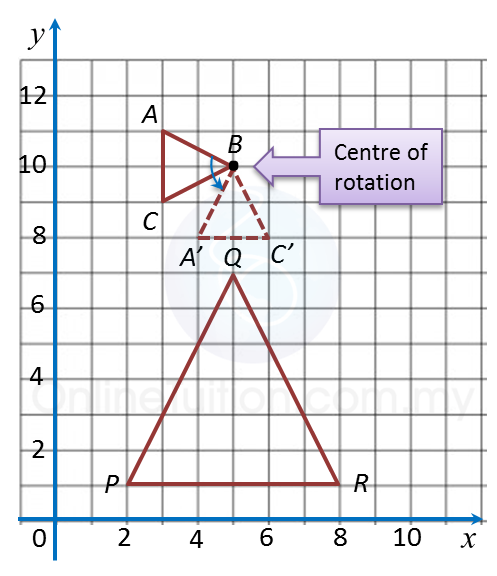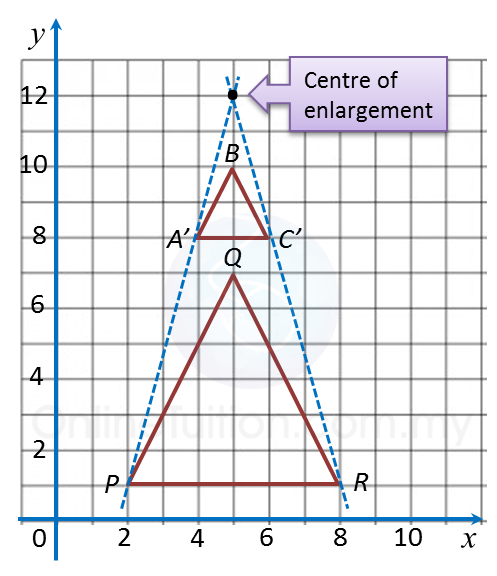# 3.2 Solving Problems involving Transformations

3.2 Solving Problems involving Transformations

Example:
The diagram below shows the triangles ABCSQT and PQR.(a) Transformation T is a translation
Transformation P is a reflection in the straight line y = 7.
State the coordinates of the image of point A under each of the following transformations:
(i) Transformation T,
(ii) Combined transformation PT.

(b)
(i) PQR is the image of triangle ABC under the combined transformations MN. Describe in full, the transformation N and the transformation M.
(ii) Given that the area of the shaded region PSTR is 200 m2, calculate the area of the triangle SQT.

Solution:
(a)(i) A(3, 11) → TA’(8, 9).
(ii) A(3, 11) → TA’(8, 9) → A”(8, 5).

(b)(i)N = Anticlockwise rotation of 90o about the point B(5, 10).

(b)(ii)= Enlargement with centre (5, 12) and a scale factor of 3.

(c)
Area of image = (Scale factor)2 x Area of object
Area of PQR = 32 x Area of SQT
Area of SQT + Area of PSTR = 9 x Area of SQT
Area of SQT + 200 = 9 x Area of SQT
200 = 9 x Area of SQT – Area of SQT
200 = 8 x Area of SQT

Area of SQT = 25 m2